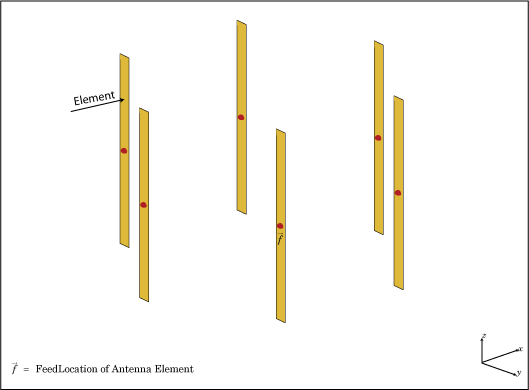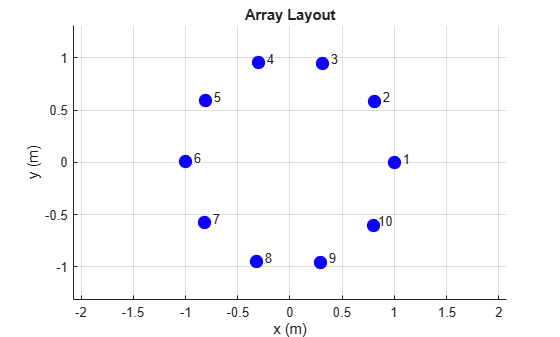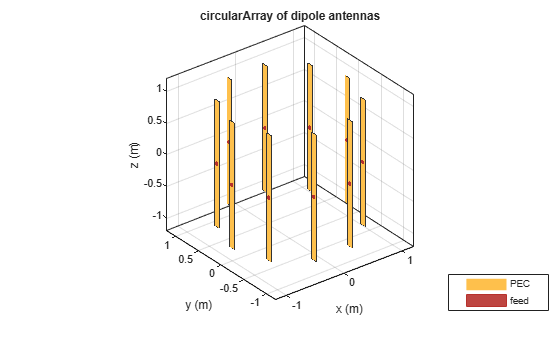Main Content

# circularArray

Create circular antenna array

## Description

The `circularArray` object is a circular antenna array. Circular array finds application in direction of arrival (DoA) systems. You can use circular arrays to perform 2-D scanning, while lowering element counts. These arrays also have the ability for 360-degree scanning. The individual elements in the circular array are part of the same array environment. This property reduces the impact of edge effects and other coupling variation.## Creation

### Syntax

``array = circularArray``
``array = circularArray(Name,Value)``

### Description

``` `array = circularArray` creates a circular antenna array in the X-Y plane. ```

example

``` `array = circularArray(Name,Value)` class to create a circular antenna array, with additional properties specified by one, or more name-value pair arguments. `Name` is the property name and `Value` is the corresponding value. You can specify several name-value pair arguments in any order as `Name1`, `Value1`,`...`, `NameN`, `ValueN`. Properties not specified retain their default values.```

## Properties

expand all

Individual antenna type, specified as a vector of objects. This property supports scalar expansion.

Example: `'Element',[monopole,monopole]`

Data Types: `char` | `string`

Number of elements in the array,specified as a positive scalar integer. The elements in the array are arranged along the X-axis.

Example: `'NumElements',4`

Data Types: `double`

Radius of array, specified as a positive scalar integer in meters.

Example: `'Radius',0.4`

Data Types: `double`

Starting angle offset for first element in array, specified as a real scalar in degrees.

Example: `'AngleOffset',8`

Data Types: `double`

Excitation amplitude for antenna elements in the array, specified as a real positive vector of size `'Element'`.

Example: `'AmplitudeTaper',[0 1]`

Data Types: `double`

Phase shift for each element in the array, specified as a real vector of size `'Element'` in degrees.

Example: `'PhaseShift',[0 2]`

Data Types: `double`

Tilt angle of the array specified as a scalar or vector with each element unit in degrees. For more information, see Rotate Antennas and Arrays.

Example: `'Tilt',90`,

Example: `'Tilt',[90 90]`,`'TiltAxis',[0 1 0;0 1 1]` tilts the array at 90 degrees about the two axes, defined by vectors.

Data Types: `double`

Tilt axis of the array, specified as:

• Three-element vectors of Cartesian coordinates in meters. In this case, each vector starts at the origin and lies along the specified points on the X-, Y-, and Z-axes.

• Two points in space, each specified as three-element vectors of Cartesian coordinates. In this case, the array rotates around the line joining the two points in space.

• A string input describing simple rotations around one of the principal axes, 'X', 'Y', or 'Z'.

For more information, see Rotate Antennas and Arrays.

Example: `'TiltAxis',[0 1 0]`

Example: `'TiltAxis',[0 0 0;0 1 0]`

Example: `array.TiltAxis = 'Z'`

Data Types: `double`

## Object Functions

 `show` Display antenna or array structure; display shape as filled patch `info` Display information about antenna or array `layout` Display array or PCB stack layout `show` Display antenna or array structure; display shape as filled patch `beamwidth` Beamwidth of antenna `charge` Charge distribution on metal or dielectric antenna or array surface `correlation` Correlation coefficient between two antennas in array `current` Current distribution on metal or dielectric antenna or array surface `design` Design prototype antenna or arrays for resonance at specified frequency `efficiency` Radiation efficiency of antenna `EHfields` Electric and magnetic fields of antennas; Embedded electric and magnetic fields of antenna element in arrays `impedance` Input impedance of antenna; scan impedance of array `mesh` Mesh properties of metal or dielectric antenna or array structure `optimize` Optimize antenna or array using SADEA optimizer `pattern` Radiation pattern and phase of antenna or array; Embedded pattern of antenna element in array `patternAzimuth` Azimuth pattern of antenna or array `patternElevation` Elevation pattern of antenna or array `returnLoss` Return loss of antenna; scan return loss of array `sparameters` S-parameter object

## Examples

collapse all

Create a circular antenna array using 10 antenna elements. View the layout of the antenna elements in the array.

`ca = circularArray('NumElements',10)`
```ca = circularArray with properties: Element: [1x1 dipole] NumElements: 10 Radius: 1 AngleOffset: 0 AmplitudeTaper: 1 PhaseShift: 0 Tilt: 0 TiltAxis: [1 0 0] ```
```figure; layout(ca)```Display the array.

```figure; show(ca)```Plot the elevation pattern of the circular array at a frequency of 70 MHz.

```figure; patternElevation(ca,70e6)```## See Also

### Topics

Introduced in R2016b

## Support

#### Hybrid Beamforming for Massive MIMO Phased Array Systems

Download the white paper